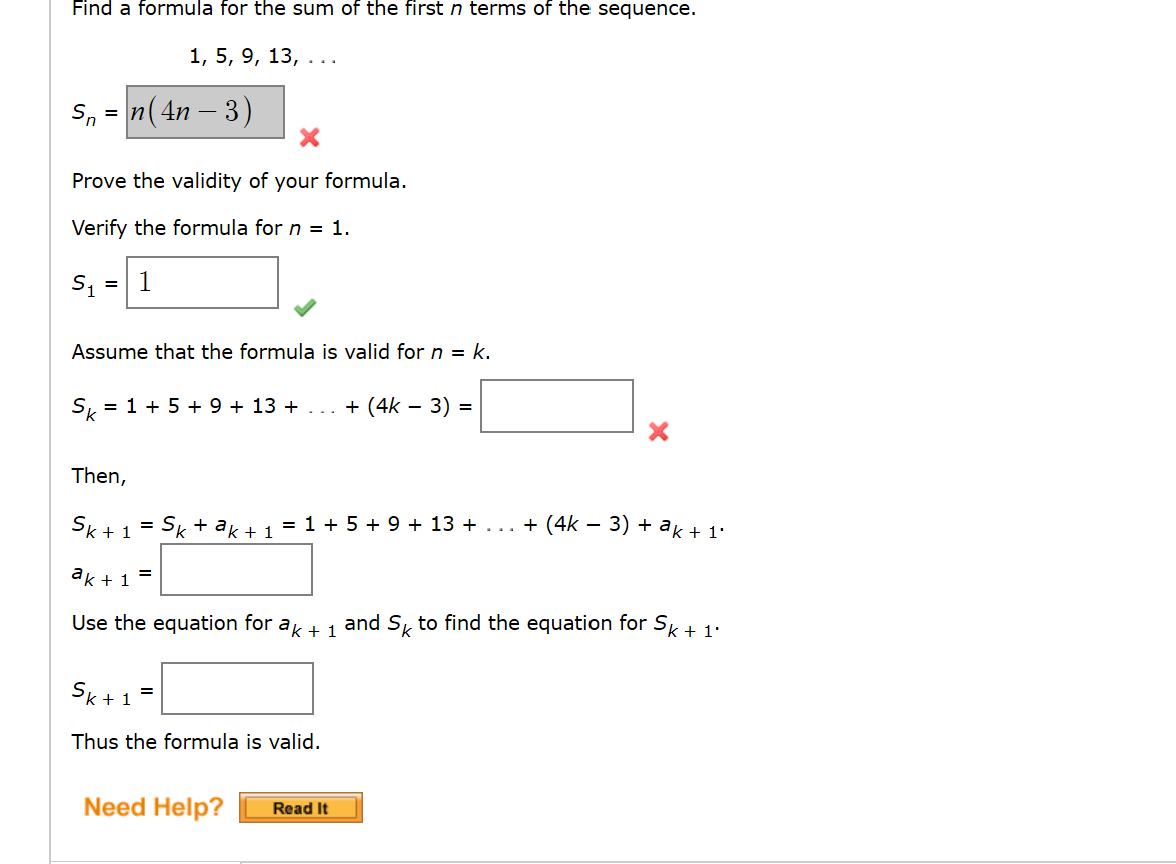# Find a formula for the sum of the first n terms of the sequence.1, 5, 9, 13,. ..Snn(4n- 3)XProve the validity of your formulaVerify the formula for n = 1.S1 1Assume that the formula is valid for n = k.(4k - 3) =S 15+ 9 13XThen,... + (4k - 3) + ak+ 1*= 1 59 + 13Sk+1Sk+ ak 1=ak1Use the equation for ak + 1and S to find the equation for Sk + 1Sk+ 1=Thus the formula is valid.Need Help?Read It

Question
7 views

I am very confused about this chapter and specifically on how the homework is breaking the problems down.

Mathematical induction is just a proof involving replacements correct?help_outlineImage TranscriptioncloseFind a formula for the sum of the first n terms of the sequence. 1, 5, 9, 13,. .. Snn(4n- 3) X Prove the validity of your formula Verify the formula for n = 1. S1 1 Assume that the formula is valid for n = k. (4k - 3) = S 15+ 9 13 X Then, ... + (4k - 3) + ak+ 1* = 1 59 + 13 Sk+1 Sk+ ak 1 = ak1 Use the equation for ak + 1 and S to find the equation for Sk + 1 Sk+ 1 = Thus the formula is valid. Need Help? Read It fullscreen
check_circle

Step 1

Given sequence is

Step 2

The first term and the difference are

Step 3

The sum of n term ...

### Want to see the full answer?

See Solution

#### Want to see this answer and more?

Solutions are written by subject experts who are available 24/7. Questions are typically answered within 1 hour.*

See Solution
*Response times may vary by subject and question.
Tagged in

### Other